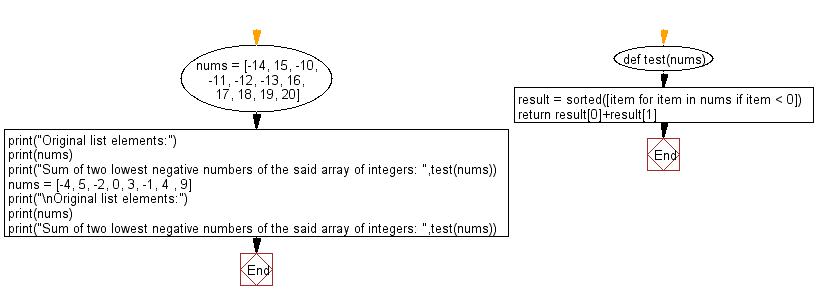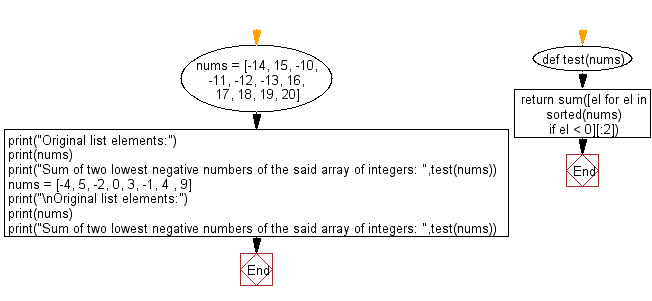﻿ Python: Sum of two lowest negative numbers of a given array of integers - w3resource# Python: Sum of two lowest negative numbers of a given array of integers

## Python List: Exercise - 213 with Solution

Write a Python program to calculate the sum of two lowest negative numbers of a given array of integers.

An integer (from the Latin integer meaning "whole") is colloquially defined as a number that can be written without a fractional component. For example, 21, 4, 0, and −2048 are integers.

Sample Solution-1:

Python Code:

``````def test(nums):
result = sorted([item for item in nums if item < 0])
return result+result

nums = [-14, 15, -10, -11, -12, -13, 16, 17, 18, 19, 20]
print("Original list elements:")
print(nums)
print("Sum of two lowest negative numbers of the said array of integers: ",test(nums))
nums = [-4, 5, -2, 0, 3, -1, 4 , 9]
print("\nOriginal list elements:")
print(nums)
print("Sum of two lowest negative numbers of the said array of integers: ",test(nums))
```
```

Sample Output:

```Original list elements:
[-14, 15, -10, -11, -12, -13, 16, 17, 18, 19, 20]
Sum of two lowest negative numbers of the said array of integers:  -27

Original list elements:
[-4, 5, -2, 0, 3, -1, 4, 9]
Sum of two lowest negative numbers of the said array of integers:  -6
```

Flowchart:## Visualize Python code execution:

The following tool visualize what the computer is doing step-by-step as it executes the said program:

Sample Solution-2:

Python Code:

``````def test(nums):
return sum([el for el in sorted(nums) if el < 0][:2])

nums = [-14, 15, -10, -11, -12, -13, 16, 17, 18, 19, 20]
print("Original list elements:")
print(nums)
print("Sum of two lowest negative numbers of the said array of integers: ",test(nums))
nums = [-4, 5, -2, 0, 3, -1, 4 , 9]
print("\nOriginal list elements:")
print(nums)
print("Sum of two lowest negative numbers of the said array of integers: ",test(nums))
```
```

Sample Output:

```Original list elements:
[-14, 15, -10, -11, -12, -13, 16, 17, 18, 19, 20]
Sum of two lowest negative numbers of the said array of integers:  -27

Original list elements:
[-4, 5, -2, 0, 3, -1, 4, 9]
Sum of two lowest negative numbers of the said array of integers:  -6
```

Flowchart:## Visualize Python code execution:

The following tool visualize what the computer is doing step-by-step as it executes the said program:

Python Code Editor:

Have another way to solve this solution? Contribute your code (and comments) through Disqus.

What is the difficulty level of this exercise?

Test your Python skills with w3resource's quiz

﻿

## Python: Tips of the Day

```print(2_000_000)
```2000000